# Assume that the three processes arrived in order: Processes                            CPU Burst Time P1  &n

Assume that the three processes arrived in order:

Processes                            CPU Burst Time

P1                                           17

P2                                            6

P3                                            8

a.     Please draw the Gantt chart if FCFS scheduling is used.

b.    Please calculate the average waiting time and average completion time under FCFS. You MUST show the calculation procedure.

c.      Please draw the Gantt chart if Round Robin is used.

d.    Please calculate the average waiting time and completion time under RR with q = 3. You MUST show the calculation procedure.

e.      Between the two CPU scheduling strategies, which one is better? Why?

Assume that the three processes arrived in order at time 0:

Processes                            CPU Burst Time

P1                                           10

P2                                            5

P3                                            7

P4                                            3

a.     If Shortest-Job-First is used, please draw the Gantt chart.

b.    Please calculate the average waiting time and average completion time under SJF.

Assume that the three processes arrived at different time:

Processes             Arrival Time               CPU Burst Time

P1                              0                                     16

P2                              2                                      8

P3                              3                                      7

P4                              4                                      4

a.     If the Shortest-Remaining-Time-First scheduling is used, please draw the Gantt chart.

b.    Please calculate the average waiting time and the average turnaround time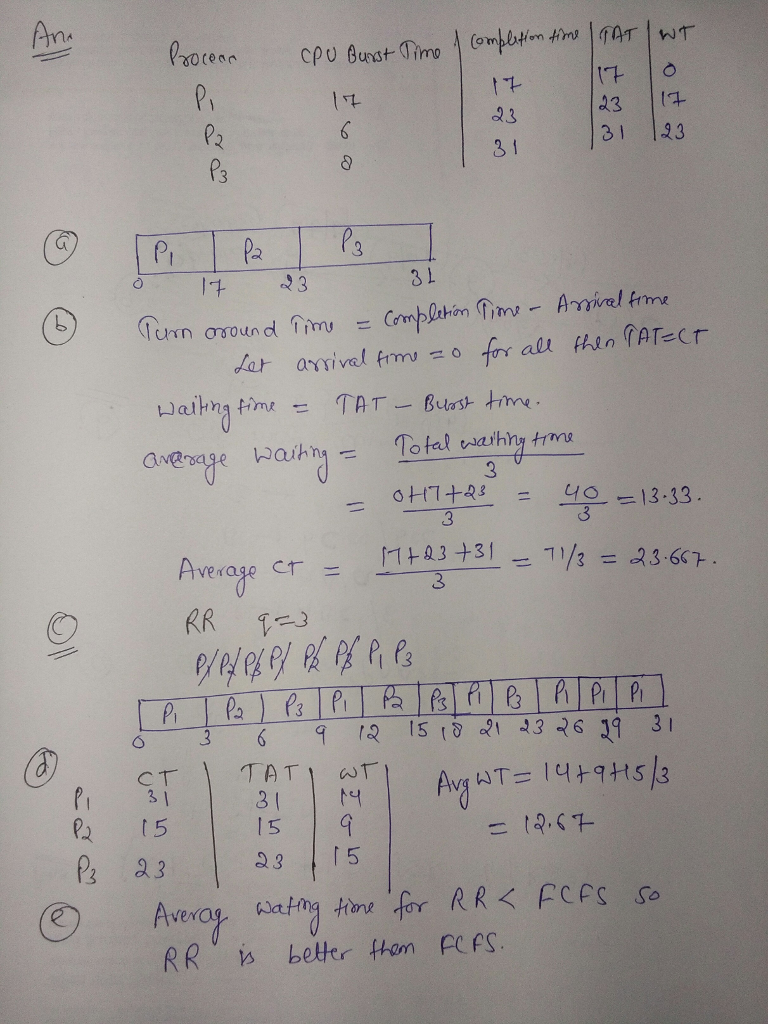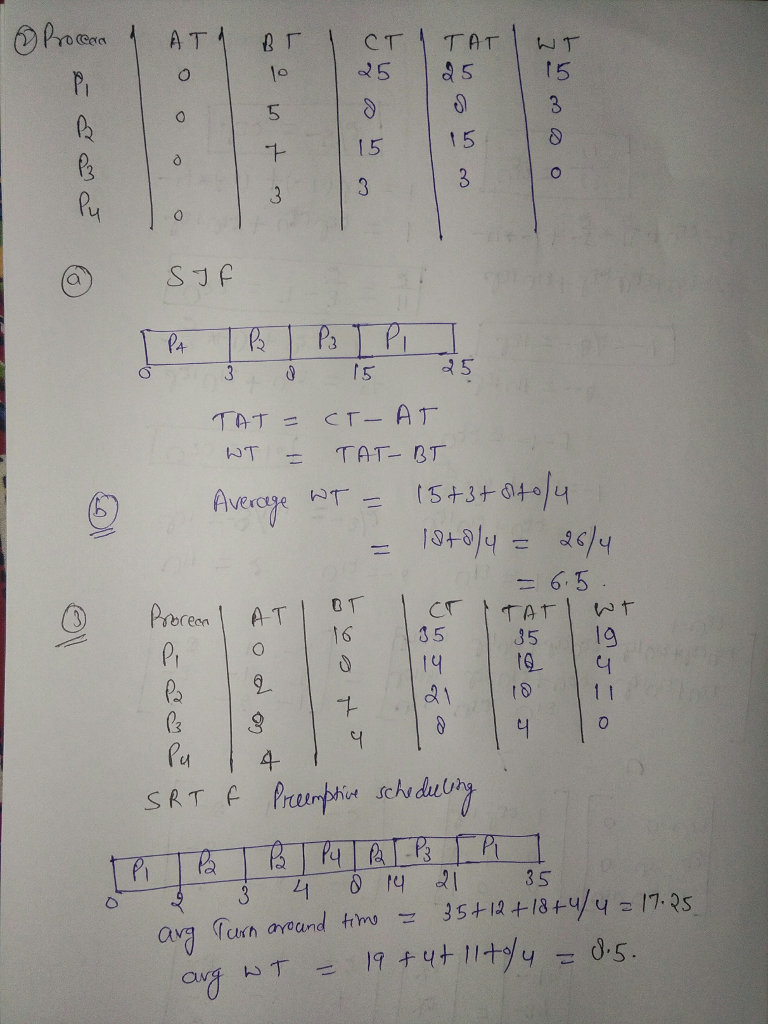#### Earn Coin

Coins can be redeemed for fabulous gifts.

Similar Homework Help Questions
• ### QUESTION 1 Consider the following set of processes, with the length of the CPU burst time...

QUESTION 1 Consider the following set of processes, with the length of the CPU burst time given in milliseconds: Process            Burst Time      Priority P1                    7                      5 P2                    2                      4 P3                    11                    3 P4                    9                      1 P5                    5                      3 The processes are assumed to have arrived in the order P1,P2, P3, P4, P5, all at time 0. a. Draw four Gantt charts that illustrate the execution of these processes using the following scheduling algorithms: FCFS, SJF, nonpreemptive priority (a smaller priority number implies a higher priority), and RR (quantum = 2). b. What...

• ### Consider the following set of processes, with the length of the CPU-burst time given in milliseconds:

Consider the following set of processes, with the length of the CPU-burst time given in milliseconds:Processburst TimePriorityP1103P211P323P414P552For each of the scheduling algorithms, FCFS, Shortest-Job-First (SJF, non-preemptive), Priority (smaller priority number implies higher scheduling priority), and RR (quantum = 1) do the following.Draw a Gantt chart to show how these processes would be scheduled.Give the turnaround time (total time from the first arrival into ready state until CPU-burst is completed) of each process.Give the waiting time (total time spent in the Ready state) of each process.Give...

• ### Computer Science Help Instructions Consider the following set of processes, with the length of the CPU-burst...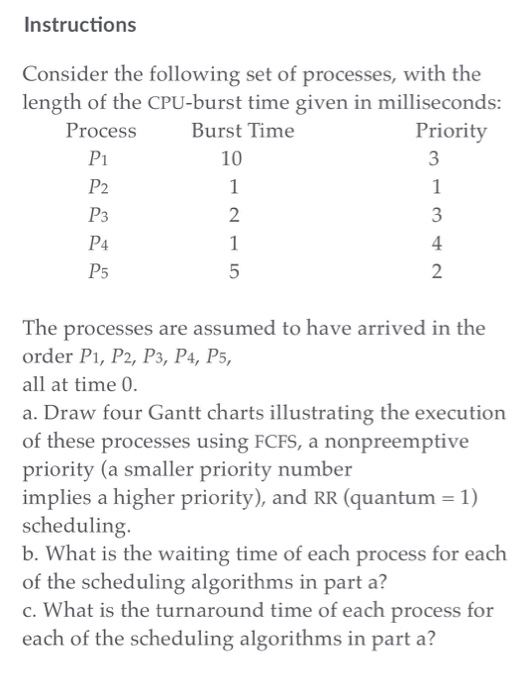Computer Science Help Instructions Consider the following set of processes, with the length of the CPU-burst time given in milliseconds: Burst Time Process P1 P2 Priority 10 4 The processes are assumed to have arrived in the order P1, P2, P3, P4, P5, all at time 0. a. Draw four Gantt charts illustrating the execution of these processes using FCFS, a nonpreemptive priority (a smaller priority number implies a higher priority), and RR (quantum 1) scheduling. b. What is the...

• ### 2. The following processes have 4 CPU burst and 3 I/O bursts each. They are all...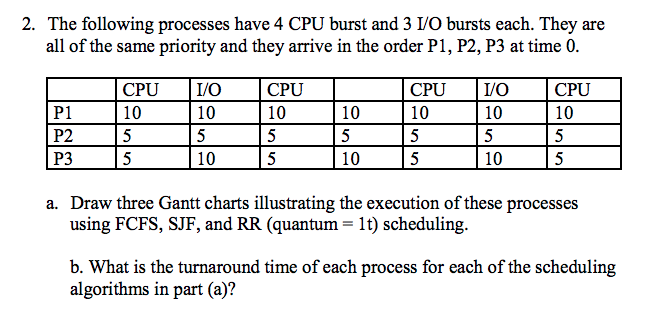2. The following processes have 4 CPU burst and 3 I/O bursts each. They are all of the same priority and they arrive in the order P1, P2, P3 at time 0 CPUO 10 CPU 10 CPUI/O 10 CPU 10 10 10 P1 P2 P3 10 10 10 a. Draw three Gantt charts illustrating the execution of these processes using FCFS, SJF, and RR (quantum- 1t) scheduling b. What is the turnaround time of each process for each of the...

• ### C language show all work 1. [50 pts] Given the following set of processes, with arrival...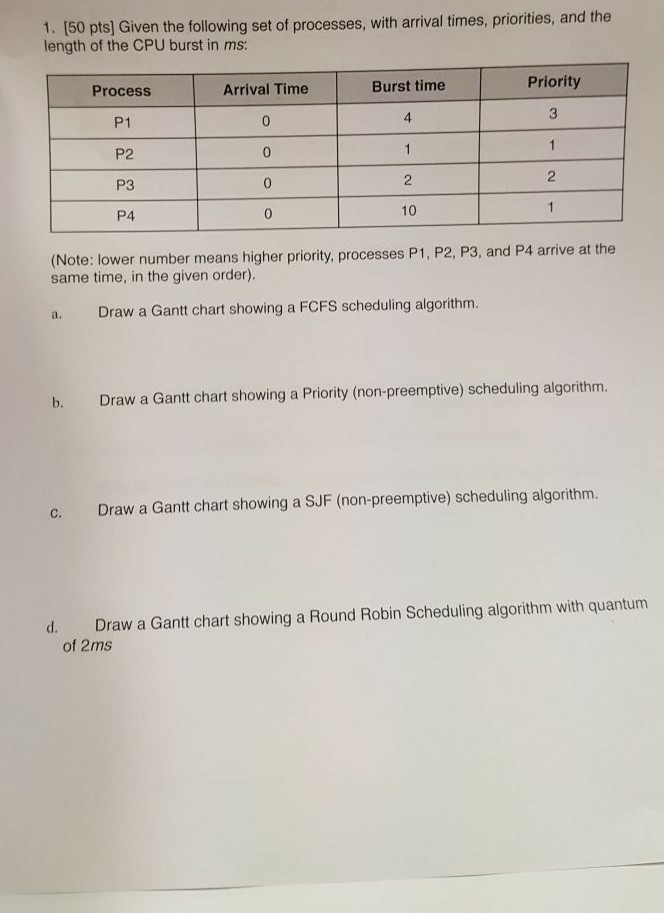C language show all work 1. [50 pts] Given the following set of processes, with arrival times, priorities, and the length of the CPU burst in ms: Priority Arrival Time Burst time Process P1 P2 P3 P4 4. 3 2 0 0 10 (Note: lower number means higher priority, processes P1, P2, P3, and P4 arrive at the same time, in the given order). a. Draw a Gantt chart showing a FCFS scheduling algorithm. b. Draw a Gantt chart showing...

• ### Consider 5 processes whose Arrival time and CPU Burst time is as follows (Process no: Arrival...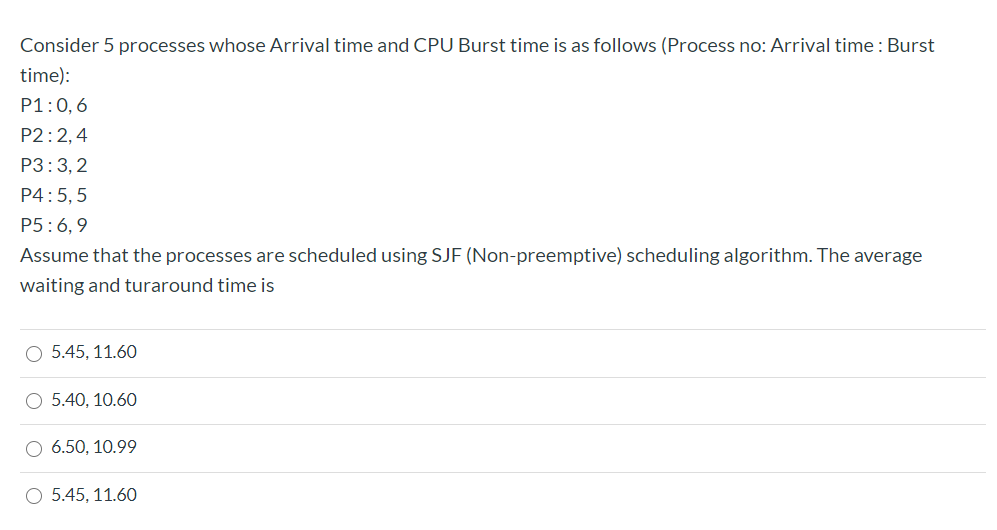Consider 5 processes whose Arrival time and CPU Burst time is as follows (Process no: Arrival time: Burst time): P1:0,6 P2:2,4 P3:3,2 P4: 5,5 P5: 6,9 Assume that the processes are scheduled using SJF (Non-preemptive) scheduling algorithm. The average waiting and turaround time is O 5.45, 11.60 O 5.40, 10.60 O 6.50, 10.99 O 5.45, 11.60

• ### Consider 5 processes whose Arrival time and CPU Burst time is as follows (Process no: Arrival...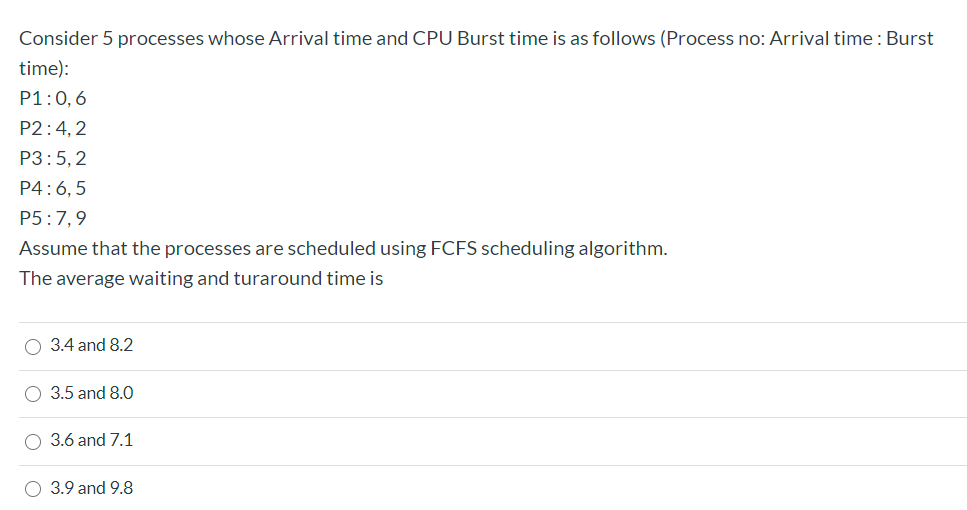Consider 5 processes whose Arrival time and CPU Burst time is as follows (Process no: Arrival time: Burst time): P1:0,6 P2:4,2 P3:5,2 P4:6,5 P5:7,9 Assume that the processes are scheduled using FCFS scheduling algorithm. The average waiting and turaround time is O 3.4 and 8.2 O 3.5 and 8.0 O 3.6 and 7.1 3.9 and 9.8

• ### V. Round Robin and SJF comparison 6 Process Arrival Time CPU Burst Time P1 0 9...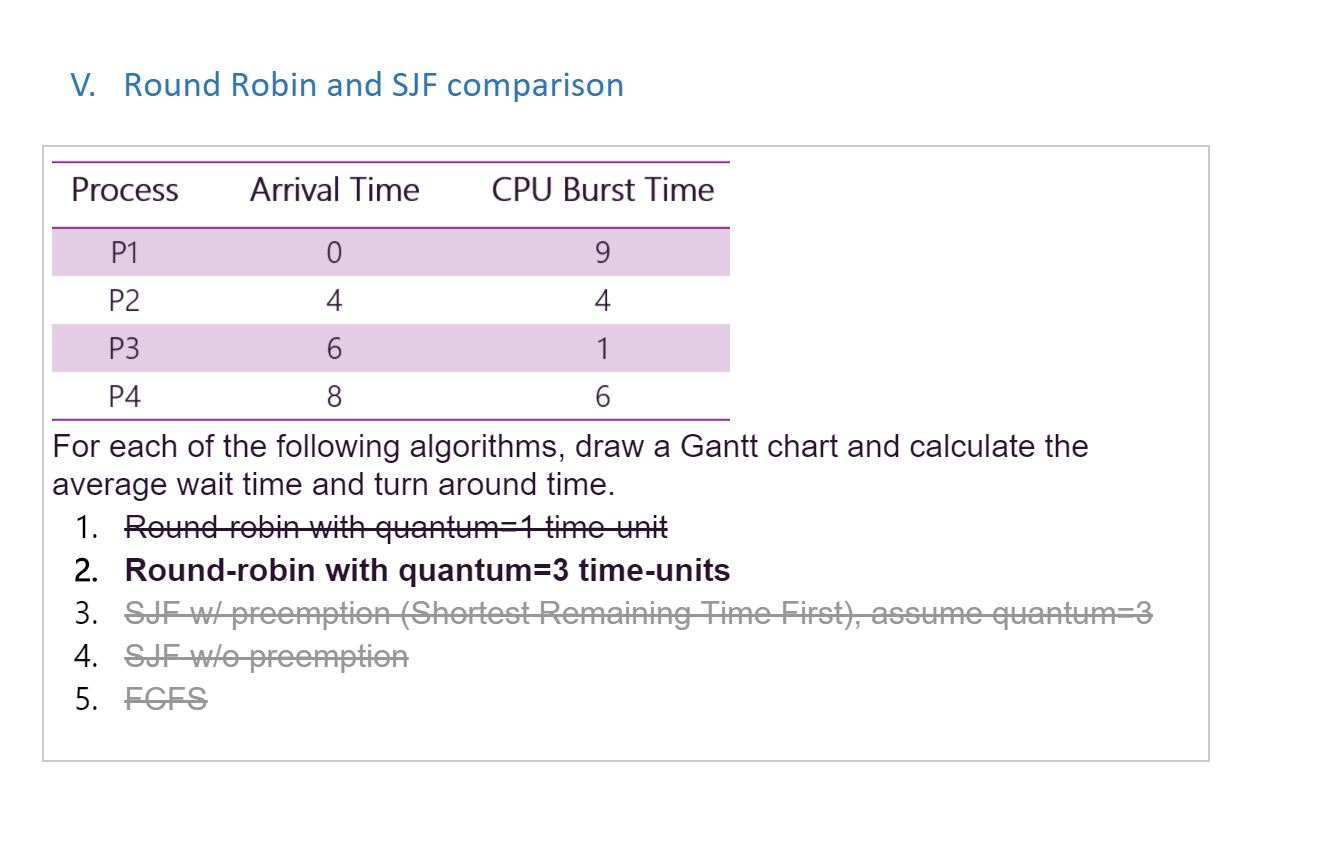V. Round Robin and SJF comparison 6 Process Arrival Time CPU Burst Time P1 0 9 P2 4 4 P3 6 P4 For each of the following algorithms, draw a Gantt chart and calculate the average wait time and turn around time. 1. Round robin with quantum=1 time unit 2. Round-robin with quantum=3 time-units 3. SJF WI preemption (Shortest Remaining Time First), assume quantum=3 4. SJE Wlo preemption 5. ECES